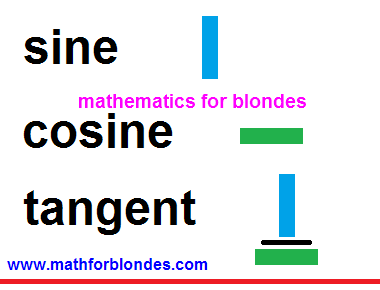## 7.02.2012

### Trigonometric functions

Trigonometric functions is simply. At the last time we considered the portrait of tangent, today we will expose the secrets of practical application of this masterpiece of painting.

Any trigonometric function is the result of division. It needs to be memorized forever. We divide something by something and as a result get a trigonometric function. That on what divided in trigonometric functions? Variants are different here. I suggest not to learn by rote you on memory all, in respect of trigonometric functions. Let us try simply to apply the portrait of tangent and minimum set of necessary knowledge.

And so, a tangent is a sine on a cosine. As we said hardly higher, in a tangent a sine is divided by a cosine. A division in mathematics can mark a fractional line or two points. We will transform our portrait of tangent in the record of partaking with two points. Here that turned out for us.Most important, what we are taught by this picture, so it to those places, where it is needed to search sines and cosines. In the system of denotations accepted by mathematicians, sines always are situated on a vertical line - it upwards-downward. Cosines always are situated on a horizontal - it to the left-right (or aside). That is a complete set will look from 6 trigonometric functions in our variant of denotations. For evidentness a sine and cosine are depicted by a different color and we will add fractional hyphens wherein without them not to do.Do not be frightened such amount of pictures. That it is represented in overhead part, above a red line, we almost learned already. We will repeat:

## a sine - it upwardsa cosine - is asidea tangent - it to divide a sine into a cosine

Do you see? The half of trigonometric functions we learned already. With the second half, that under a red line, yet simpler - invert that you learned already heels over head. Remaining trigonometric functions are shots reverse already known to us. We swap a numerator and denominator - all is ready already for us.

With a cotangent all is simple. A cotangent is this attitude of cosine toward a sine. With gimbleting of tangent no problems arise up - he has both a numerator and denominator. But as to be with a sine and cosine? We do not have denominators of these shots in fact! Do not worry, any number has a denominator that in mathematics, writing is not accepted is unit. In a secant and cosecant exactly this unit appears in a numerator, and a cosine and sine are written in a denominator.

It is important to memorize! In the pairs of the names of trigonometric functions a secant is a cosine and cosecant - a sine can be only one prefix to on two names. If we take a sine for basis, then a reverse shot will be named cosecant. If we take a cosine, then a reverse shot is named secant, because one prefix to we already used up on a cosine.

It is important to memorize! In the pairs of the names of trigonometric functions a secant - is a cosine and cosecant - a sine can be only one prefix co on two names. If we take a sine for basis, then a reverse shot will be named cosecant. If we take a cosine, then a reverse shot is named secant, because one prefix co we already used up on a cosine.

In principle, it fully sufficiently, in determinations of trigonometric functions to feel, close as an oyster in water. A question remains not found out, that at a sine and cosine to write in a denominator instead of unit. In fact we said in beginning, that trigonometric functions are a result of division(relation) or shot. Do not worry, mathematicians will prompt us, that exactly they want there to see. For this purpose there are determinations of trigonometric functions. We will talk next time about them.

1.1.